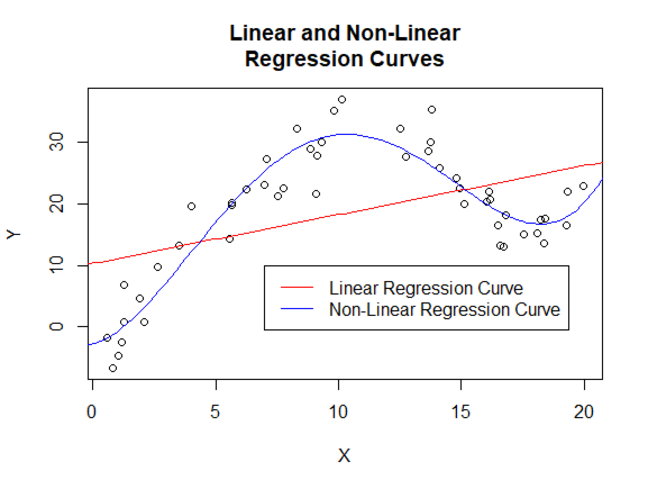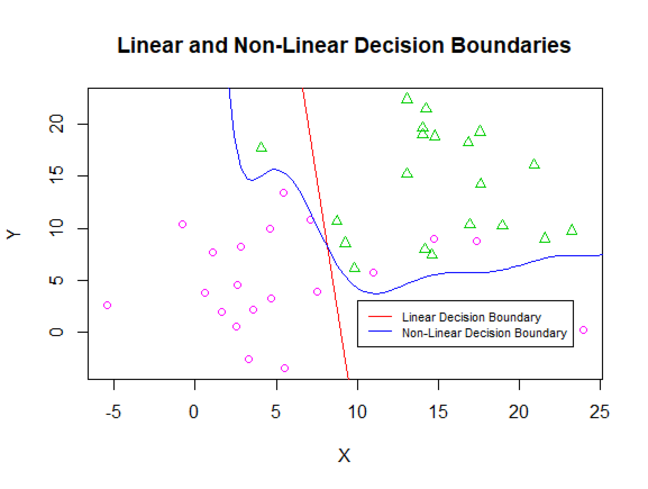## Want to keep learning?

This content is taken from the The Open University & Persontyle's online course, Advanced Machine Learning. Join the course to learn more.
1.6

# Types of Learning

## Supervised Learning

In supervised learning, we seek to learn a statistical model capable of providing good estimates of the values of a (set of) target variable(s) from a set of input features. This learning is undertaken using labelled training data, which is to say that the values of the target variable are known in the data used to fit the parameters of the model.

Key characteristics:

• Data contains input features (X) and target (Y) variables
• Training data is labelled (values of target variables known)
• Model seeks to estimate values of target variables based on input features
• Accuracy of the model can be evaluated by its performance on new cases

We will look at a number of supervised learning techniques in weeks 2 and 3.

### Regression & Classification

An important distinction within supervised learning is between regression and classification tasks. The difference between the two is the type of target variable (we write this assuming only one such target variable though there can be many).

The target variable in the regression case is a real number. The aim is either to obtain a point estimate (a single value) of the target variable given the values taken by the input features, or a probability distribution over the values of the target variable given the same. Associated with regression models is the concept of a the regression curve (or surface/hypersurface in higher dimensions), which gives an estimated value of the target variable for each combination of input features.In the classification case, the target variable is a discrete or nominal variable. This means it takes one of a number of classes as a value. The aim is to either obtain an estimate of the value taken by the target variable given the values of the input features, or a probability distribution of the target variable given the same. Associated with classification models is the concept of a decision boundary, which is a curve (surface/hypersurface) that separates the feature space into regions where the target variable is estimated to take different values.The feature space is the mathematical space form by taking the values of the input features as coordinate axes.

There are other types of variables, such as integer and ordinal variables. Integer valued variables are numeric variables which only take integer values. Ordinal variables, like nominal variables, take one of a number of classes as values. However in the ordinal case there is an ordering on the values taken. This ordering is not numeric, in the sense that it makes no sense to talk of ratios between different values. There are some statistical models that deal specifically with both integer and ordinal target variables, but they are relatively few and not covered in this course.

Additionally there are complex valued variables. Many regression models can handle such variables, but we will not explicitly discuss them in this course.

## Unsupervised Learning

In unsupervised learning, our statistical models are used to describe patterns found in the data. The data used in unlabelled, which means there is no division in it between target and input variables. Examples include clustering, anomaly detection and explicit or implicit density estimation. In many cases, such as clustering or anomaly detection, the accuracy of the classification provided by the model is difficult or impossible to independently verify.

Key characteristics:

• Variables not differentiated into input features and target variables.
• Training data is unlabelled (no such thing as target variables)
• Model seeks to identify some pattern in the data
• Accuracy of the model may be difficult or impossible to independently verify.

We will look at a number of unsupervised learning techniques in week 3.

## Semi-supervised Learning

Semi-supervised learning pursues the same goal as supervised learning, but makes use not only of labelled training data, where the values of the target variable are known, but also unlabelled training data, where the values of the target variable are unknown. Typically the amount of unlabelled data is substantially larger than the amount of labelled data.

Key characteristics:

• Data contains input features (X) and target (Y) variables
• Training data includes both labelled (values of target variables known) and unlabelled (values of target variables unknown) cases
• Model seeks to estimate values of target variables based on input features
• Accuracy of the model can be evaluated by its performance on new labelled cases, though these may be difficult to obtain.

We will look at a single semi-supervised learning technique in the final week of this course.

## Reinforcement Learning

Reinforcement learning shares the same goal as supervised learning, in that some target variable should be estimated from a set of input features. However, like unsupervised learning, the true value of this target variable is not present in available data. Instead, during the training process, guesses about the value of the target variable for given inputs will lead to some form of feedback from the environment - not in the form of directly indicating the correctness of the guess, but rather in the sense of some reward or punishment related to the correctness of the guess. Typically, the process of data acquisition is, in at least some degree, controlled by the algorithm itself.

An example is a model learning the best moves to make under different circumstances in an arcade game. The model is never informed that a particular move was optimal under particular circumstances, but is given feedback about sequences of moves via the game score.

Key characteristics:

• Data contains only input features (X)
• Training data is unlabelled (values of target variables unknown)
• Model seeks to estimate values of target variables based on input features
• Estimates about the target variable lead to some form of feedback from the environment
• Training typically online and data acquisition during training commonly under at least partial control of the model or training process.
• Performance of the model can typically be evaluated only in terms of the environmental feedback

We will look at a simple reinforcement learning technique in the final week of this course.